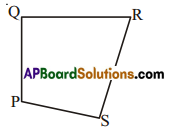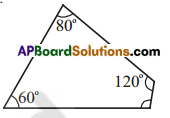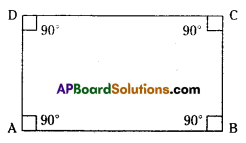# HSSlive: Plus One & Plus Two Notes & Solutions for Kerala State Board

## Wednesday, October 19, 2022AP Board Class 7 Maths Chapter 12 Quadrilaterals Ex 1 Textbook Solutions PDF: Download Andhra Pradesh Board STD 7th Maths Chapter 12 Quadrilaterals Ex 1 Book Answers

## Andhra Pradesh State Board Class 7th Maths Chapter 12 Quadrilaterals Ex 1 Books Solutions

 Board AP Board Materials Textbook Solutions/Guide Format DOC/PDF Class 7th Subject Maths Chapters Maths Chapter 12 Quadrilaterals Ex 1 Provider Hsslive

3. Look for your Andhra Pradesh Board STD 7th Maths Chapter 12 Quadrilaterals Ex 1 Textbooks PDF.

Find below the list of all AP Board Class 7th Maths Chapter 12 Quadrilaterals Ex 1 Textbook Solutions for PDF’s for you to download and prepare for the upcoming exams:

Question 1.
(i) Name the sides, angles, vertices and diagonals.
(ii) Also name all the pairs of adjacent sides, adjacent angles, opposite sides and opposite angles. .Solution:
sides = PQ, QR, RS and SP
angles = ∠P, ∠Q, ∠R, and ∠S
vertices = P, Q, R and S
diagonals = PR and QS
ii) Pairs of adjacent sides = (PQ, QR), (QR. RS), (RS, SP), (SP, PQ)
Pairs of adjacent angles (∠P, ∠Q). (∠Q, ∠R), (∠R, ∠S), (∠S, ∠P)
Pairs of opposite sides = (PQ, RS) and (QR, PS)
Pairs of opposite angles = (∠P,∠R) and (∠Q, ∠S)

Question 2.
The three angles of a quadrilateral are 60°, 80° and 1200. Find the fourth angle?
Solution:
Given three angles = 60°,80°,120°
Sum of the given three angles = 60°+ 80° + 120° = 260°
Sum of the four Interior angles of a quadrilateral = 360°
∴ The fourth angle = 360° — 260° = 100°

Question 3.
The angles of a quadrilateral are in the ratio 2 : 3 : 4: 6. Find the measure of each of the four angles.
Solution:Given that ratio of the four angles = 2 : 3 : 4 : 6
Sum of the terms in the ratio = 2 + 3 + 4 + 6 = 15
Sum of the four angles = 360°
∴ 1st angle = 215 x 360° = 48°
2nd angle = 315 x 360° = 72°
3rd angle = 415 x 360° = 96°
4th angle = 615 x 360° = 144°

Question 4.
Find each of them.
Solution:Sum of the four angles in a quadrilateral = 360°
Each of the angle = 360∘4 = 90°
∠A = ∠B = ∠C = ∠D = 90°

Question 5.
In a quadrilateral, the angles arex°, (x + l0)°, (i+ 20)°, (x + 30)°. Find the angles.
Solution:
Given angles are x°, (x + 10)°, (x + 20)°, (x + 30)°
Sum of the four angles = x + x + 10° + x + 20° + x + 30° = 4x + 60°
But the sum of the four angles = 360°
4 x 60° = 60° = 360°
4x = 360° – 60° = 300°
x = 300∘4 = 75°
∴ The angles are x = 750
x + 10°- 75°+ 10° = 85°
x + 20° = 75° +20° = 95°
x + 30° = 75° + 30° = 105°

Question 6.
The angles of a quadrilateral cannot be in the ratio 1: 2 : 3 : 6. Why? Give reasons.
(Hint: Try to draw a rough diagram of this quadrilateral)
Solution:
Given that the angles of a quadrilateral cant be in the ratio 1: 2 : 3 : 6
If the ratio is 1 : 2 : 3 : 6 then
the sum of terms of ratio = I + 2 + 3 + 6 = 12
Sum of the angles = 360°
∴ 1st angle = 112 x 360° = 30°
2nd angle = 212x 360° = 60°
3rd angle = 312 x 360° = 90°
4th angle = 312 x 360° = I80
i.e., 4th angle is 180°, a straight angle.
A quadrIlateral cant he formed with these angles.

## Andhra Pradesh Board Class 7th Maths Chapter 12 Quadrilaterals Ex 1 Textbooks for Exam Preparations

Andhra Pradesh Board Class 7th Maths Chapter 12 Quadrilaterals Ex 1 Textbook Solutions can be of great help in your Andhra Pradesh Board Class 7th Maths Chapter 12 Quadrilaterals Ex 1 exam preparation. The AP Board STD 7th Maths Chapter 12 Quadrilaterals Ex 1 Textbooks study material, used with the English medium textbooks, can help you complete the entire Class 7th Maths Chapter 12 Quadrilaterals Ex 1 Books State Board syllabus with maximum efficiency.

## FAQs Regarding Andhra Pradesh Board Class 7th Maths Chapter 12 Quadrilaterals Ex 1 Textbook Solutions

#### Can we get a Andhra Pradesh State Board Book PDF for all Classes?

Yes you can get Andhra Pradesh Board Text Book PDF for all classes using the links provided in the above article.